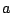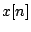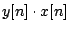Next: Frequency Up: Sinusoids, amplitude and frequency Previous: Units of Amplitude   Contents   Index

# Controlling Amplitude

Perhaps the most frequently used operation on electronic sounds is to change their amplitudes. For example, a simple strategy for synthesizing sounds is by combining sinusoids, which can be generated by evaluating the formula on Page, sample by sample. But the sinusoid has a constant nominal amplitude, and we would like to be able to vary that in time.In general, to multiply the amplitude of a signalby a factor, you can just multiply each sample by, giving a new signal. Any measurement of the RMS or peak amplitude ofwill be greater or less by the factor. More generally, you can change the amplitude by an amountwhich varies sample by sample. Ifis nonnegative and if it varies slowly enough, the amplitude of the product(in a fixed window fromto) will be that of, multiplied by the value ofin the window (which we assume doesn't change much over thesamples in the window).

In the more general case where bothandare allowed to take negative and positive values and/or to change quickly, the effect of multiplying them can't be described as simply changing the amplitude of one of them; this is considered later in Chapter 5.Next: Frequency Up: Sinusoids, amplitude and frequency Previous: Units of Amplitude   Contents   Index
Miller Puckette 2006-12-30